## 3.19Calculated derivatives

The introduction to matrix construction, Sec. 3.5 , described implicit and explicit discretisation of terms in an equation. It concluded that the principal derivatives of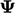that can be treated implicitly — forming matrix coeﬃcients in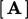— are a time derivative, advection and Laplacian.

Terms with other derivatives must be calculated from respective ﬁelds, e.g.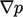from current values of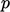. In Sec. 3.15 , we have described discretisation of a gradient, which is always explicit. This section gathers together the other derivatives found in equations for ﬂuid dynamics and associated models.

### General divergence term

A general divergence term is any term that can be represented by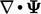. It excludes the Laplacian term which includes a gradient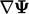, and advection which includes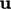.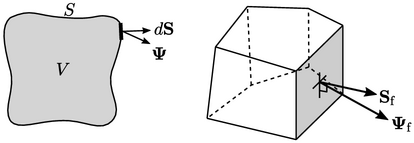The discretisation of a general divergence term is an explicit calculation using current values of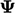. It is based on a surface integral using the divergence deﬁnition in Sec. 2.23 as shown below: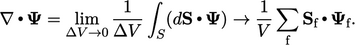(3.30)
The face value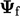is generally interpolated from cell values using the linear scheme. Terms discretised using this scheme include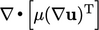in Eq. (2.45 ), a divergence of stress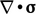, etc.

### Curl of a vector

The curl derivative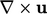is calculated from the gradient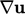and applying the Hodge dual operator given by Eq. (2.40 ) using the following relation: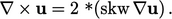(3.31)
In other words,is discretised according to a scheme from Sec. 3.15 , from which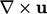is calculated by Eq. (3.31 ).

A derivative which appears in some model equations is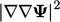, described as “mag-square grad-grad”. This derivative returns a scalar since the mag-square, e.g.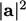, represents the inner product of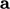with itself, as shown in Eq. (2.7 ).
The mag-square calculation always uses the appropriate inner product to reduce the result to a scalar. For a tensor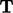, it is the double inner product, i.e.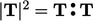.
The grad-grad operator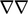yields a third-rank tensor in the case that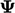is a vector ﬁeld. To avoid storing third-rank tensors, the mag-square grad-grad operator is evaluated by summing the result from the operator on each componentofby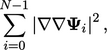(3.32)
where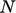is the number of components in.